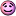CodeGuru Home VC++ / MFC / C++ .NET / C# Visual Basic VB Forums Developer.com

1.Junior MemberJoin Date
Jan 2009
Posts
16

Hey guys,

I can't seem to get the declaration for overloading the [] operator correctly.

Code:
`friend const int& operator[] (unsigned int argInt);`
Code:
```const int& operator[] (unsigned int argInt){
if (getDegree() > argInt){
return 0;
}else{
for (int i = 0; i < nCoeff; ++i){
if (degrees[i] == argInt){
return coeff[i];
}
}
}
}```
 I also tried const int& Polynomial:perator[] but it gave me an error saying 'binary '[' : 'Polynomial' does not define this operator or a conversion to a type acceptable to the predefined operator'

First off my syntax for overloading this operator is incorrect but I do not know where.

Also it can't find getDegree() nCoeff, degrees and coeff - these are variables (and one function) that are available in the class but because I have no reference to the object - I don't know how to access the object.

When overloading other operators ++ I have access to the object - is there a way I can access the object in the [] operator?

Lang
Last edited by Lang; February 28th, 2009 at 04:32 PM.Reply With Quote

2.Elite Member Power PosterJoin Date
Apr 1999
Posts
27,449

I also tried const int& Polynomial:perator[] but it gave me an error saying 'binary '[' : 'Polynomial' does not define this operator or a conversion to a type acceptable to the predefined operator'
What is "Polynomial"?

Please post the exact code that duplicates the error.

http://www.parashift.com/c++-faq-lit...t.html#faq-5.8Originally Posted by LangI can't seem to get the declaration for overloading the [] operator correctly.
First, there should be two overloads of operator [] -- one that's const and one that isn't .

http://www.parashift.com/c++-faq-lit...html#faq-18.12

Regards,

Paul McKenzieReply With Quote

3.Junior MemberJoin Date
Jan 2009
Posts
16

Polynomial is a class I have created.

Polynomial is represented by an array of integers in variable coeff - when someone calls
Polynomial[index] it will return the integer associated with x^index - so the return type is int

Code:
```const int operator[] (unsigned index){
if (getDegree() > argInt){
return 0;
}else{
for (int i = 0; i < nCoeff; ++i){
if (degrees[i] == argInt){
return coeff[i];
}
}
}
}```
This is the same code as in my original post - because its the code that generates the error.

I get 'operator []' must be a non-static member' as the error

Thanks,
Lang
Last edited by Lang; February 28th, 2009 at 09:22 PM.Reply With Quote

4.Elite Member Power PosterJoin Date
Apr 1999
Posts
27,449Originally Posted by LangPolynomial is a class I have created.
I know you created it. The point of me asking and giving you the "how to post" link is that there is no code posted that shows this class.

When you get a compiler error, it is required you show the code that is in error in its full context. This way, all we have to do is copy the code into our compiler, compile it, see the error ourselves, and give you the answer.

If it's simple enough, maybe we can spot the error without compiling. But a compiler error that tells us about classes we can't see is not helpful.

Regards,

Paul McKenzieReply With Quote

5.Junior MemberJoin Date
Jan 2009
Posts
16

EDIT: Ok I figured it out - I called (*poly2) -- now all that remains is how to fix my class so that calling poly works correctly

Ok, here is class Polynomial with all 3 of its constructors. This file is classes.h

Code:
```class Polynomial{
int *coeff; //array of coefficients
int nCoeff;

public:
//Polynomial with constant
Polynomial(const int a = 0);
//Polynomial degree n, array(n + 1)
Polynomial(const int, int *);
//copy constructor
Polynomial(const Polynomial &);
//deconstructor
~Polynomial();

//getDegree returns degree of polynomial
const int getDegree();

int operator[](unsigned int index);
};

/*
CONSTRUCTORS
*/
Polynomial::Polynomial(const int a){
coeff = new int;
coeff = a;
nCoeff = 1;
}

Polynomial::Polynomial(const int a, int *c){
nCoeff = a + 1;
coeff = new int[a+1];

for (int i = 0; i < (a+1); ++i){
coeff[i] = c[i];
}
}

Polynomial::Polynomial(const Polynomial &p){
//they're not the same
if (this != &p){
delete []coeff;
coeff = p.coeff;
nCoeff = p.nCoeff;
}
}

/*
GETDEGREE()
*/
const int Polynomial::getDegree(){
int i = nCoeff - 1;
while (coeff[i] == 0) --i;
return i;
}

//array operator
int Polynomial::operator[](unsigned int index){
return coeff[index];
}```
And here is the main procedure for testing:

Code:
```#include <iostream>
#include <cstdlib>
#include <conio.h>
#include "classes.h"

using namespace std;

int main(){
int coeff = {1, 3};
int coefft = {6, 2};
Polynomial * poly = new Polynomial(1, coefft);
Polynomial * poly2 = new Polynomial(1, coeff);
cout << *poly2; //*** line
delete poly;
delete poly2;
return 0;
}```
*** line gives me an error of illegal indirection - the issue I'm having is if I take the * out of *poly I get an access violation when getDegree() is called - as a matter of fact I have no idea why getDegree is even being called!
Last edited by Lang; March 4th, 2009 at 11:01 AM.Reply With Quote

6.Elite Member Power PosterJoin Date
Jan 2006
Location
Singapore
Posts
6,768

Try:
Code:
`cout << (*poly2);`Reply With Quote

array, operators, overload, overloading####Posting Permissions

• You may not post new threads
• You may not post replies
• You may not post attachments
• You may not edit your posts
•